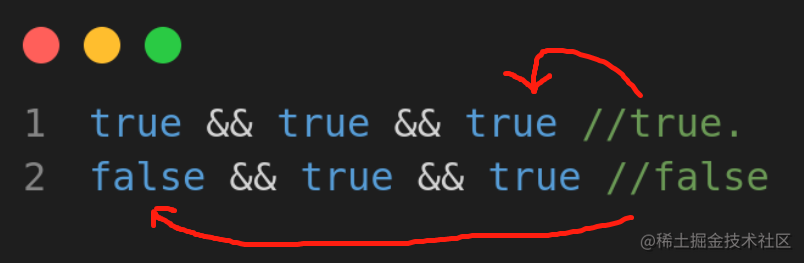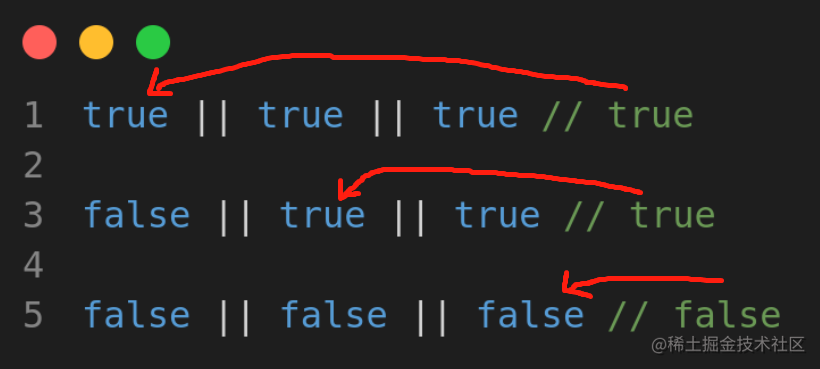# 在精不在多，3 分钟看 3 个 JS 小把戏## trick 1 : 两组逻辑与或

``````let a="one",b="two",c="three";

let d = a && b && c;

console.log(d)

``````// "three"

``````let a = "",b="two",c="three";

let d = a && b && c;

console.log(d)

`````` // ""

``````let a="one",b="two",c="three";

let d = a || b || c;

console.log(d)

`````` // "one"

``````let a = "",b="two",c="three";

let d = a || b || c;

console.log(d)

``````  // "two"

• AND (&&)• OR (||)## trick 2 : 将变量设为 key

``````var stu_address = 'address';
var student = {
name:'mick',
age : 10,

}
// {name:'mick', age : 10, stu_address : 'chennai'}

“trick 登场” ！

``````var stu_address = 'address';
var student = {
name:'mick',
age : 10,

}

//{name: 'mick', age: 10, address: 'chennai'}

## trick 3 : 数据和对象转换

``````let arr = [1,2,3,4,5,6,7,8,9]

const convert_obj = {...arr};
// {"0": 1, "1": 2, "2": 3, "3": 4, "4": 5, "5": 6, "6": 7, "7": 8, "8": 9,"9": 10}

``````let obj = {
one : 'a',
two : 'b',
three : 'c'
};

/*1. Object.keys*/

const keys = Object.keys(obj) //['one', 'two', 'three']

/*2. Object.values*/

const values = Object.values(obj) // ['a', 'b', 'c']

/*3. Object.entries*/

const entries = Object.entries(obj) // [ ["one", "a"], ["two", "b"], ["three","c"] ]

OK，以上便是本篇分享，希望能有所帮助~